Ch 6. Entropy Multimedia Engineering Thermodynamics Entropy Tds Relations EntropyChange IsentropicProcess IsentropicEfficiency EntropyBalance (1) EntropyBalance (2) ReversibleWork
 Chapter 1. Basics 2. Pure Substances 3. First Law 4. Energy Analysis 5. Second Law 6. Entropy 7. Exergy Analysis 8. Gas Power Cyc 9. Brayton Cycle 10. Rankine Cycle Appendix Basic Math Units Thermo Tables Search eBooks Dynamics Fluids Math Mechanics Statics Thermodynamics Author(s): Meirong Huang Kurt Gramoll ©Kurt GramollTHERMODYNAMICS - CASE STUDY SOLUTION A salesperson states that this spot cooler can work at the atmospheric pressure without any energy consumption. Evaluate his claim. Assumptions: Air is an ideal gas and has constant specific heats. The flow is steady, no work and heat transfer involved. Kinetic and potential energy changes are ignored.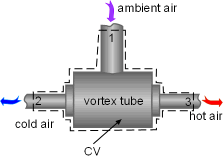Control Volume of Spot Cooler Take the spot cooler as a control volume shown on the left. If the spot cooler satisfies both the first and the second law of thermodynamics, it will work. To verify if it satisfies the first law, check the energy balance of the system. To verify if it satisfies the second law, check the entropy change of the entire process. With all the assumptions, the mass and energy balance of the control volume are: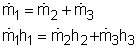The mass balance is satisfied since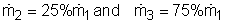The constant-specific-heat assumption is also used. So with constant specific heats, the energy balance becomes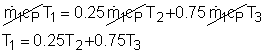Substituting the temperatures into both sides of the above equation yields       right side = 0.25(24 + 273) +0.75(40 + 273) = 309       left side = 36 + 273 = 309 = right side The result above shows the energy balance is satisfied. To check if the spot cooler satisfies the second law, calculate the entropy change of the entire process. If the entropy change is not negative, the process can happen. With constant specific heats, the entropy change equals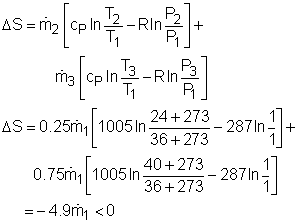The result shows that the spot cooler cannot work at atmospheric pressure. The air at the inlet needs to be compressed to a higher pressure to make the cooler work, which requires energy input.